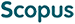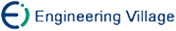周俊萍 姜蕴晖 殷明浩. 最坏情况下X2SAT问题的上界[J]. 计算机研究与发展, 2014, 51(3): 598-605.
 引用本文: 周俊萍 姜蕴晖 殷明浩. 最坏情况下X2SAT问题的上界[J]. 计算机研究与发展, 2014, 51(3): 598-605.Zhou Junping, Jiang Yunhui, and Yin Minghao. New Worst-Case Upper Bounds for X2SAT[J]. Journal of Computer Research and Development, 2014, 51(3): 598-605.
 Citation: Zhou Junping, Jiang Yunhui, and Yin Minghao. New Worst-Case Upper Bounds for X2SAT[J]. Journal of Computer Research and Development, 2014, 51(3): 598-605.## New Worst-Case Upper Bounds for X2SAT

• 摘要: 最坏情况下XSAT问题上界的研究已成为一个热门的研究领域.针对XSAT的泛化问题X2SAT提出了算法X2SAT-N,该算法首先利用简化算法Simplify对公式进行化简,然后通过分支树的方法对不同情况的子句进行分支.证明了该算法可以将X2SAT问题的时间复杂度由目前最好的O(1.4511n)提高到O(1.4203n),其中n为X2SAT公式中变量的数目.X2SAT问题实例的大小不仅依赖于变量的数目还依赖于公式的长度,时间复杂性是根据问题实例的大小所组成的函数计算所得.因此又提出了算法X2SAT-L,并从公式长度的角度证明了X2SAT问题在O(1.3643l)时间上界内可解.

Abstract: The problem of X2SAT is a generalized problem of XSAT. Given a conjunctive normal function, this problem asks whether there exists a satisfying assignment for the input formula, such that exactly two literals in each clause are true. The rigorous theoretical analysis of the algorithms for solving X2SAT problem is proposed in the literature. An algorithm X2SAT-N based on Davis-Putnam-Logemann-Loveland (DPLL) is first presented to solve the X2SAT problem. In the algorithm X2SAT-N, a simplification process is first called to simplify the input formula. After that, several strategies are used to cut the branches on different kinds of clauses. It can be proved that the worst-case upper bound of algorithm X2SAT-N for solving X2SAT should be O(1.4203n), which improves the previous fastest X2SAT algorithm of O(1.4511n) up to now. Here n denotes the number of variables in a formula. Additionally, an algorithm called as X2SAT-L for solving X2SAT problem is also presented. This algorithm is also based on DPLL and using similar simplification process. We prove that the worst-case upper bound of this algorithm is O(1.3643l), where l is the length of the formula. Within our knowledge, this algorithm is the best algorithm for X2SAT with the parameter of the length of the formula./下载:  全尺寸图片 幻灯片
• 分享
• 用微信扫码二维码

分享至好友和朋友圈